telescopeѲptics.net          ▪▪▪▪                                             CONTENTS

# 4.7.1. Secondary spectrum and spherochromatism

Secondary spectrum

Fortunately, chromatic aberration of a single lens - primary spectrum - can be significantly reduced with a pair of lenses combined. Defining "achromatic" as the level of correction where both longitudinal and lateral chromatism are cancelled for two wavelengths near the opposite ends of the visual range, a lens pair - or "doublet" - is achromatic if the individual lens focal lengths are the reciprocal of their Abbe numbers: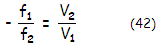with f1,2 being the lens focal lengths (given by Eq. 1.1.) in the e-line, and V1,2 their respective Abbe numbers (dispersion), with the subscripts 1 and 2 referring to the front and rear lens, respectively. The equation implies that the two lenses need to have opposite powers, and that the weaker lens (normally, the negative element of a telescope objective) needs to have proportionally stronger dispersion in order to offset chromatism induced by the stronger lens.

For near-contact or contact pairs, the system focal length f relates to the focal lengths of individual lenses f1 and f2 as 1/f=(1/f1)+(1/f2). This determines the individual lens' focal lengths in terms of the system focal length and Abbe numbers as: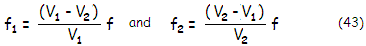If we choose to cancel chromatism for the  F (486nm blue) and C (656 nm red) lines, then the curvatures of the front and rear lens need to relate as: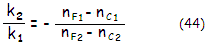with k=(1/R1)-(1/R2), where R1,2 is the front and rear lens radius of curvature, respectively,  and nF1, nC1 and nF2, nC2 refractive indici of the selected distant wavelengths for the front and rear lens, respectively. This relation tells that for bringing F and C to a common focus, the magnitude of dispersive powers of two glasses in F and C for two glasses needs to relate as the inverse of their optical power (since the curvature k is directly proportional to the optical power). For instance, if the positive element in a doublet has 50% greater optical power than the negative element, its dispersive differential between F and C has to be as much smaller.

In other words, secondary spectrum of a doublet does not depend on the lens shape, only lens power. This leaves enough room for lens "bending", so that other (monochromatic) aberrations are also corrected or minimized. But bringing two widely separated wavelengths to a common focus does not make all the wavelengths over the visual range nearly parfocal. Those in between will still focus shorter, and those closer to the range ends will focus longer. This form of residual chromatic defocus is called secondary spectrum.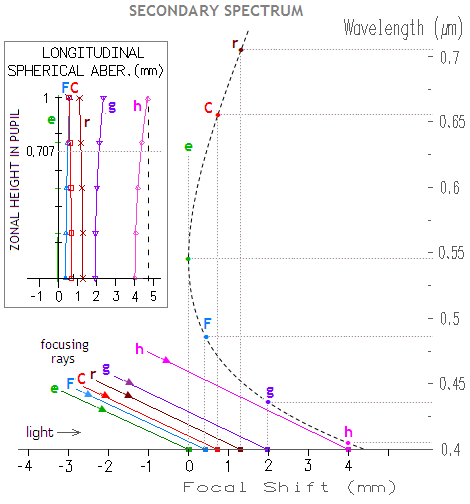Graph at left shows secondary spectrum of a 100mm aperture f/10 doublet achromat with a common crown/flint combination (BK7/F2). With F and C line focusing close together, the shortest focus is around the e-line, with all other wavelengths focusing longer. If the longitudinal foci of individual wavelengths are projected onto a vertical wavelength scale, they form a parabolic curve (dashed) which represents focal (chromatic) shift vs. wavelength. As graph shows, all other wavelengths also form common focus, with every wavelength toward the blue end having its counterpart toward red. Height of the parabola's tip (and its shape, slightly) changes with the particular mode of correction. The standard mode brings together F and C foci, because at the time of early achromats it was believed that eye sensitivity for these two lines are similar, and bordering significant. In fact, the blue is more significant even in photopic (bright light) conditions, and more so toward lower light conditions, typical of those during observing. Hence the optimum correction for general observing would bring together F and a wavelength somewhat shorter than C, tilting the parabola somewhat

Since only a single point on axis - paraxial focus, formed by paraxial (central) rays - represents a wavelength here, this graph does not show spherical aberration - i.e. change in longitudinal focus location with the zonal height - present in each wavelength (spherochromatism). For that, chromatic defocus needs to be shown vs. zonal height in the pupil (inset top left); for instance, marginal focus for the violet h line is about 0.75mm longer than its paraxial focus, with the corresponding P-V error of spherochromatism at the best focus (0.707 zone focus location for primary spherical aberration) given by 0.75/64F2=0.0001172mm, or 0.29λ for λ=0.000405mm wavelength. For near-equal wavefront error, the common F/C focus is close to the marginal rays foci of these two wavelengths, with the paraxial foci somewhat separated, as indicated by the focal shift plot

In order for a doublet to have identical focal length for an additional, third wavelength in the mid-range (e-line here), it also needs to satisfy the relation: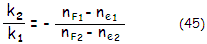which, considering Eq. 44, implies the equality:

(nF1-ne1)/(nF1-nC1)=(nF2-ne2)/(nF2-nC2)          (45.1)

i.e. that the value of the ratio of dispersive differential between F and e vs. that in F and C needs to be identical for the two glasses in order to have these three wavelengths brought to a common focus.

The (nF -ne)/(nF -nC) factor is called relative partial dispersion (RPD) of glass for these three wavelengths, hereafter denoted PF,e. General formula for the relative partial dispersion is Px,y=(nx-ny)/(nF-nC). It determines the magnitude of separation between the common F/C paraxial focus, and paraxial focus of the wavelength x, as:

Δf = [P(x,y)2-P(x,y)2]f/(V1-V2) = (ΔP/ΔV)f

where Δf is the secondary spectrum, f is the objective focal length, subscripts 1 and 2 denote the two glasses, and ΔP and ΔV are the RPD and Abbe number differential for the glasses, respectively. As the relation implies, two glasses of equal RPD for given three wavelengths will have zero focus differential for those wavelengths - i.e. will bring them to a common focus - while with non-zero RPD differential the magnitude of defocus between the wavelength x and common F/C focus, as a fraction of the wavelength, is proportional to the ratio ΔP/ΔV. Hence, two glasses with equal  PF,e values will bring F, C and e spectral lines to a common focus. What is commonly referred to as secondary spectrum is the Δf value for these three wavelengths, i.e. the e-line focus vs. common F/C focus separation; however, it could also be any other wavelength vs. F/C focus. For instance, using Pg,F and corresponding wavelengths will indicate separation between the violet g-line and common F/C focus.

One should keep in mind that this is strictly valid for the paraxial foci. When spherical aberration in non-optimized wavelengths (spherochromatism) is significant, their best focus shifts significantly away from their paraxial focus, which to a smaller or larger extent makes the actual chromatism differ from one based on the paraxial foci.

Thus doublet satisfying Eq. 42-45 brings two widely separated wavelengths and a third, mid-wavelength, to a common focus. Practically, assuming negligible spherochromatism, it means that all other wavelengths within this range are also nearly parfocal. Such doublet is called apochromatic. Thus, apochromatic objective corrects for both, primary and secondary color error. That alone still doesn't guarantee high-level correction of chromatism, since spherochromatism in lens objectives with strongly curved surfaces can be significant. This is why Abbe's apochromatic condition, in addition to practically eliminating secondary spectrum, requires good correction of spherical aberration across the wavelength range (tertiary spectrum) as well, with the most stringent (not specified, though) requirement being for the optimized wavelength.

When only achromatic condition (Eq. 44) is satisfied, two widely separated wavelengths come to a common focus, with the the longitudinal focus deviation for the wavelengths between them increasing relatively slowly toward approximately the mid-wavelength - green e-line focus for the common F/C focus - and much faster toward external wavelengths, more so toward blue/violet than red. Longitudinal focus deviation of these other wavelengths vs. common focus is called secondary spectrum. It is commonly expressed as the longitudinal separation between the common-focus wavelengths (blue/red) and shortest-focus (green) focal length, with the latter being the mid-wavelength with the maximum defocus relative to the common focus. For the canonical F/C common focus, the farthest-focusing mid-wavelength focus is (approximately) that for the e-line.

Since, according to Eq. 1.2-1.2.1 their respective focal lengths can be written as:

1/fFC=(nF1-1)k1+(nF2-1)k2, and

1/fe=(ne1-1)k1+(ne2-1)k2,

the differential, or F/C-e secondary spectrum, is

1/fFC-1/fe=Δ/f=(nF1-ne1)k1+(nF2-ne2)k2        (45.2)

Obviously, we can have secondary spectrum cancelled by making k2/k1=(nF1-ne1)/(nF2-ne2), but we cannot do it unless we already have fulfilled k2/k1=(nF1-nC1)/(nF2-nC2), which is the condition for the primary goal of bringing two wavelengths near the opposite ends of visual spectrum to a common focus. Without it, it would mean simply bringing F and e lines, alone, to a common focus.

It is good to make distinction between the terms shortest-focus and optimized wavelength, since the two may and may not be the same. As mentioned, the former refers to the mid wavelength with the maximum longitudinal separation from the common focus of two widely separated wavelengths. The latter refers to the wavelength with minimized spherical aberration (since only a single wavelength can have it cancelled). Graphically, the former is the wavelength with paraxial focus at the largest longitudinal separation from the paraxial common focus (FIG. 67 bottom), i.e. the one at the tip of the chromatic focal shift plot. For the canonical F/C common focus, the shortest-focusing wavelength is approximately the e-line. On the other hand, optimized wavelength is the one with nearly straight longitudinal zonal plot (e-line on FIG. 67 top), regardless on its position on the chromatic focal shift plot. By a slight adjustment in one of the inner radii and/or separation, any wavelength can be optimized to near-zero spherical aberration.

If, for instance, the canonical F/C common focus achromat is overcorrected in the e-line - i.e. has this line curving to the right on the longitudinal aberration plot - optimized wavelength is shifted toward the red end, to the wavelength which is somewhat less than 1/4 wave undercorrected (inversely proportional to the wavelength) when the e-line is, as it should be, the one with the minimum spherical aberration, while the error of overcorrection is added (again, at a somewhat larger scale, increasing in proportion to the wavelength) toward the blue/violet end.

This directly implies that for all but one wavelength, there is an inherent axial error in an achromat, and that is spherical aberration (spherochromatism), increasing away from the optimized wavelength; at any wavelength, defocus error exists only relative to some other wavelength, and can be cancelled by refocusing.

The respective curvatures of the two lens elements k1=(1/R1)-(1/R2) and k2=(1/R3)-(1/R4), can be expressed as k1=1/(nF1-nC1)(V1-V2)f and  k2=1/(nF2-nC2)(V2-V1)f. After substitution, axial separation between the red/blue (F/C) focus and the e-line focus - the secondary spectrum - is given by:For the canonical achromat with the common C/F focus and the shortest e-line focus (so called C-e-F correction), using BK7/F3 glasses for the front and rear lens, respectively, this gives longitudinal chromatic error (as the separation of the green focus from the common blue/red focus, a standard measure of secondary spectrum) as:

Δf = - (3.348732 - 4.5799n1 + 2.230453n2)f             (46.1)

with n1 and n2 being the indici of refraction for the chosen wavelength for the front and rear lens, respectively, and f the system focal length (since P(F,e)1<P(F,e)2, secondary spectrum is numerically negative, because it is measured from the common F/C focus, which is farther away from the objective, to the right).

Substituting indici for the e-line (n1=1.51872, n2=1.61685 for BK7/F3 doublet)  in the above relation gives Δf=-0.000546f, or -f/1832 as the separation between the green (e-line) and red/blue focus (the minus sign indicating that the latter is farther away from the objective). When measured from d-line focus, which is closer to the common F/C focus, secondary spectrum is slightly better than f/2000; the difference is, of course, only numerical, since the level of chromatic correction doesn't change.

Alternately, since the plot of chromatic defocus vs. shortest-focus wavelength within the visual range (0.4-0.7μm) nearly conforms to a parabola, nearly identical in shape and coordinates for the common achromat glass pairs, secondary spectrum in this range, as the defocus relative to the focus of the wavelength's that has the shortest focus (tip of the parabola), can be closely approximated by a parabolic formula of the form:

y = x2/(2x-0.5)           (47)

where y is the defocus measured from the tip of parabola (the shortest-focus wavelength), and x=λ0A is the wavelength differential in microns between the shortest-focus (λ0) and arbitrary (λA) wavelength.

Thus, any two different wavelengths λ1 and λ2 with non-zero wavelength differential vs. shortest-focus (zero x) wavelength will have their respective defocus relative to the zero-defocus wavelength (tip of parabola) relating as:

y1/y2=(2x2-0.5)x12/(2x1-0.5)x22.

Knowing that the actual defocus for the F/C lines vs. zero-defocus e-line wavelength (reversed secondary spectrum) in the standard achromat is yF,C= ΔfF,C=f/1850, f being the focal length, and their respective wavelength differential in microns are -0.06 and 0.11, substituting these into (y1/y2)ΔfF,C gives the defocus ΔfA for an arbitrary wavelength within the visual range as:

ΔfA = [106xA2/(2xA-0.5)]ΔfF,C  for the (numerically positive) wavelength differential xA toward blue/violet, and

ΔfA = [60xA2/(2xA-0.5)]ΔfF,C  for the (numerically negative) wavelength differential xA toward red.

From the nominal defocus ΔfA it is easy to obtain the corresponding P-V defocus wavefront error from W=ΔfA/8F2, F being the objective's focal ratio. For ΔfA in mm, the wavefront error in units of the wavelength λ is Ww=ΔfA/8λF2, with λ also in mm. Obviously, when F and C line foci coincide, the shorter wavelength will have (assuming negligible spherochromatism, i.e. pure defocus error), proportionally larger wavefront error.

Although nearly coinciding F and C-line focus is canonically assumed, this is not the best chromatic correction mode, since eye sensitivity in the F-line is more than twice that in the C-line even in photopic mode (bright light conditions), and still increasing significantly toward scotopic (dim light conditions) mode. Thus better correction mode is with the wavefront error in F and C nearly equalized, or even somewhat smaller in the blue line. The significance of the difference between these two modes increases with achromat's relative aperture (FIG. 67).FIG. 67: Longitudinal zonal aberration for F, e and C lines (top) and chromatic shift plot (bottom) for the canonical common-F/C-focus and equal wavefront error (WFE) correction mode. TOP: Each outlined plot shows the canonical secondary spectrum mode, with the paraxial F/C foci coinciding. However, better chromatic correction is achieved with the wavefront error in F and C nearly equalized, due to the lower error in the blue/violet. In a 100mm f/10 achromat, due to the presence of spherochromatism, the wavefront errors in F and C are nearly equalized when their marginal rays nearly coincide, with the blue paraxial focus closer to the green focus, with the C paraxial focus nearly twice farther away than that in F; in a 100mm f/5, approximately when 0.8 zone foci in F and C nearly coincide, with the C paraxial focus about five times farther away from the paraxial e-line focus than the F focus, and in a 100mm f/15 when F and C focusing segments are separated, with the paraxial C focus about 50% farther away from the green than the blue F focus. Dashed horizontal line indicates the location of best (diffraction) focus, with the wavelengths focusing at it being at the intersection with the chromatic shift parabola. BOTTOM: Chromatic shift plots corresponding to the longitudinal zonal aberration plots above show the effect of switching from the canonical common F/C focus to the generally better equal F/C WFE as a shift of the plot to the left, toward shorter wavelengths (expected, since it results in lowering the error in the F line, and increasing it in the red). The shift is approximated with 248/F3/2 in nanometers, or 0.25/F3/2 in microns. Since the rate of change with any given sideways shift of the plot is significantly higher at its raised (blue/violet) end, switching to the equal F/C WFE mode results in a better overall chromatic correction; the gain is most significant at f/5, with the polychromatic Strehl (430-670nm, photopic) SP increasing from 0.53 to 0.63, still appreciable at f/10 (0.79 vs. 0.76), and negligible at f/15. The new common focus shifts from F and C to somewhat shorter wavelengths (intersect of the black curve and dotted gray). Obviously, this plot shift to the left also results in the shortest-focus wavelength shift toward shorter wavelengths. It is negligible at f/15 and f/10, but at f/5 it can be significant: here, the plot also sinks slightly below the zero line, which means that some shorter wavelengths now focus shorter than the e-line (the apparent plot sag is due to the selected primary wavelength in raytrace being e-line, with respect to which is the horizontal line drawn). Specifically, the shortest focus is in the 524nm wavelength, bending back toward  506nm, where it coincides with the optimized e-line focus (however, since spherical aberration can be corrected only in a single wavelength, there is as much as 1/4 wave p-v at 506nm's best focus, and four times as much at its paraxial focus, nearly coinciding with the best e-line focus). The best diffraction focus is shifted somewhat from e-line toward the common blue/red focus (dashed). Note that the focal shift scale for the three f-ratios is not uniform.

These plots imply that chromatic error in an achromat with given glass combination and optical quality (including spherical aberration level) will vary somewhat with its secondary spectrum mode; the faster objective, the more so. The defocus value for the equal F/C error mode can be approximated using the same expression as for the common F/C focus mode, only with the wavelength differential xA replaced by (xA-0.25/F3/2), keeping in mind that xA is positive for the wavelengths shorter than e-line, and negative for the longer ones.

Following table shows numerical values of axial defocus (secondary spectrum) and corresponding P-V wavefront error in two actual objectives, for the five common spectral lines spanning the visual range (note that the wavefront error is a subject of both, longitudinal defocus and spherical aberration).

 100mm f/10 BK7/F2 ACHROMAT PARAXIAL DEFOCUS (P-V WFE) SP h  405 g 436 F 486 e 546 C 656 r 707 paraxial F/C foci coinciding Δf 5.45 2.7 0.55 0 0.55 1.1 0.76 Ww 14.8 7 1.62 0 0.88 1.73 near-equal wavefront error Δf 4.06 1.93 0.41 0 0.75 1.36 0.79 Ww 13 5.9 1.2 0 1.2 2.1

The near-equal F/C wavefront error secondary spectrum mode does not strictly define the best mode of chromatic correction. According to raytrace, polychromatic Strehl for photopic eye is at its maximum for the error in F nearly 20% smaller than in C, i.e. with their respective paraxial foci somewhat more separated. However, considering eye's shift toward mesopic mode in the typical observing session, the F error - and that in the blue-violet end in general - should be still smaller relative to the C error, more so in observing relatively dim objects.

Note that this assumes no appreciable spherochromatism; even moderate level of spherical aberration in any given wavelength spreads its focus over a longitudinal segment, with the best focus location shifted away from the Gaussian (paraxial) focus, which is the basis of measurement for the secondary spectrum. Moderate spherochromatism can either somewhat reduce, or increase the actual chromatic error (defocus and spherical aberration combined); significant amounts are generally undesirable.

Although a term secondary spectrum generally applies to all wavelengths deviating from the common red/blue focus, it is most often used to designate the above focal separation, i.e. that between the common blue/red focus and focus of the shortest-focus (green) wavelength, normally also optimized for the minimum spherical aberration. Since the defocus for other wavelengths is nearly proportional to the F/C-to-e separation, it is a measure of the magnitude of secondary spectrum. The limit to chromatic correction for doublet achromat made with common glasses, expressed as the red/blue-to-green focal separation is somewhat smaller than f/1800 if measured as F/C-to-e-line foci separation, and ~f/2000 when measured as F/C-to-d-line foci separation. Centered on e-line, defocus increases exponentially (with the square of wavelength differential) toward either end of the spectrum (FIG. 68).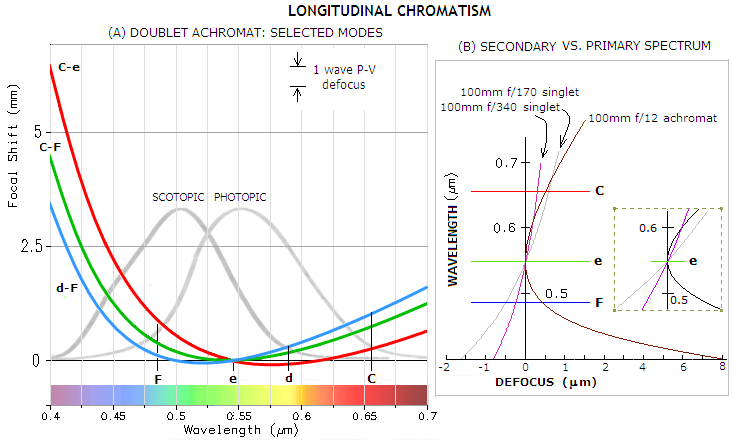FIGURE 68
: LEFT: Secondary spectrum in a 100mm
f/10 doublet achromat. Canonical doublet achromat brings blue F-line and red C-line focus close together, with the shortest-focus being that of the green e-line (C-F correction). Spherical aberration is canceled at the e-line. Since C- and F-line are brought to a common focus, their axial separation from the e-line focus (focal shift) is near-identical, an so are their respective blurs (which, considering smaller Airy disc at the shorter wavelength, implies somewhat larger wavefront error in the blue). Defocus aberration worsens much more rapidly toward the blue end of the spectrum. This is even more pronounced in C-e corrected achromats, where C- and e-line are brought to the common focus, with the shortest focus shifting closer to the d-line, and with reduced defocus error in the red. However, the blue/violet end is practically sacrificed, fading away into much more quickly expanding chromatic defocus. The downside is not only in losing the blue/violet end, but also in compromising a portion of the green toward the blue. Considering eye sensitivity curves for bright (photopic) and dim (scotopic) light conditions, correction shifted toward the blue end (d-F) offers best overall visual performance. As mentioned before, these standard chromatic shift plots show the paraxial focus shift, hence they don't show the common foci on the same horizontal line (it is the best foci of these respective lines that are near each other, i.e. approximately at the same horizontal line). Note that the small differences in the height of the tip of parabola are due to raytrace placing zero at the default wavelength, in this case the
e-line.
RIGHT: Proper combination of two glasses of different dispersions not only greatly reduces longitudinal chromatism, it also changes the form of aberration. While that in a single lens (primary chromatism) has shorter wavelengths focusing shorter, and longer wavelengths longer, the combined lens - an achromat - has all wavelengths but one in the mid-range focusing farther away. Similarly to the singlet, the rate of defocus increase with the wavelength differential is significantly higher toward shorter wavelengths. However, while defocus error increase with wavelength differential is closer to the linear in the singlet, in the achromat it changes exponentially (averaged over blue/violet and orange/red, with the square of wavelength differential but, as the graph shows, significantly faster in the former), effectively forming a parabola tilted toward the longer wavelengths. Consequently, when the defocus error of a singlet in F and C is at the similar level to that in a doublet (100m
f/170 singlet vs. 100mm f/12 achromat above), the former still has significantly more chromatism (0.55 vs. 0.80 polychromatic visual Strehl), due to its error in all the wavelengths between F and C, except the e-line, being larger. A singlet needs to have the F/C error nearly half that of the achromat (100mm f/340 vs. 100mm f/12 achromat) in order to reach its chromatism level.

Since the sum of the relative partial dispersions for an apohromatic doublet is, by definition, effectively zero, so is its secondary spectrum. In reality, there is always some residual secondary spectrum, but it is entirely negligible with well designed and made objectives.

Since it is the combination of optical power and dispersion properties of two glasses that determines degree of chromatic defocus, it can't be significantly improved by adding more lens elements of identical or similar glass types. Triplet objective makes it easier to have better overall correction of aberrations, but will not have appreciably lower secondary spectrum than a doublet made of the same two glass types. The most significant advantage of a triplet objective is that it allows for significantly weaker lens surfaces and, consequently, greatly reduces spherochromatism. That makes it objective type of choice for well corrected faster/larger refracting objectives. In addition, if instead of a single common glass type it uses two different ones, neither has to have near-zero relative partial dispersion (RPD) differential vs. low-dispersion element, as long as their respective RPD are opposite in sign, and nearly offsetting one another. That widens the number of possible glass combinations available for a triplet vs. doublet, making finding the glasses easier.

Spherochromatism

Unlike the magnitude of secondary spectrum, which can be quickly found from the RPD differential, or estimated just from looking at the RPD plot, chromatic spherical aberration or, spherochromatism (tertiary spectrum), is more difficult to anticipate. It changes not only with the refractive index (wavelength) but also with both, surface curvature and lens shape, requiring more extensive calculation. More so because its higher-order form (secondary spherical) can be significant, particularly in relatively fast apochromatic doublets.

As mentioned, lower-order (primary) spherical aberration can't be made zero in a single lens, but can be cancelled in a doublet. However, it can be cancelled only for a single wavelength. Others wavelength will be uncorrected, the farther away from the optimum wavelength, the more so.

From Eq. 7, the wavefront error of primary spherical aberration of a thin lens contact doublet at the best (diffraction) focus can be expressed as:

W = (S1+S2)(ρ4-ρ2)             (48)

with S1 and S2 being the peak aberration coefficients of the front and rear lens, respectively, and ρ the ray height in the pupil (aperture) in units of the radius. The peak aberration coefficients are S1=s1d4 and S2=s2d4, with s1 and s2 being aberration coefficients for the front and rear lens, respectively, and d the pupil (aperture) radius. General expression for the aberration coefficient of spherical aberration of a thin lens is:with n being the refractive index, q=(R2+R1)/(R2-R1) the lens shape factor, and p=1-(2f/i) the lens position factor (FIG. 69), with f being the lens focal length, and i the lens-to-image separation.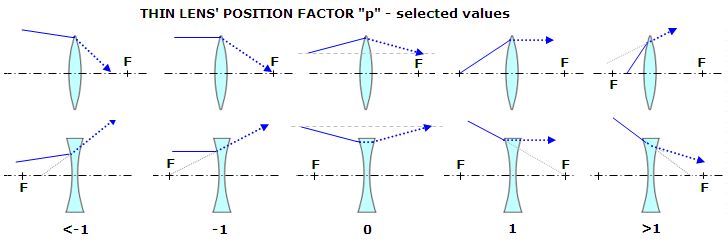FIGURE 69: Position factor p of a thin lens changes with the lens type (positive/negative) and the properties of light cone incident to it, with respect to lens' focal point  F and the resulting image separation i, as given by p=1-2f/i.

For the front lens the object is at infinity, so i1=f1 and p1=-1, resulting in: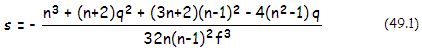For the rear lens, the final image separation equals doublet's focal length, thus i2=fd and p2=1-(2f2/fd), with f2 being the rear lens focal length, and fd the doublet focal length. The thin lens' focal length f is given by f=R1R2/(n-1)(R2-R1). Calculated values for p2 and q2 are substituted in Eq. 49 to obtain the rear lens aberration coefficient s2.

For cancelled spherical aberration, the sum of aberration coefficients for the front and rear lens must be zero. This can be achieved only for a single value of the refractive index n, that is, for a single wavelength. In a doublet achromat with spherical aberration cancelled for the e-line, the blue end of spectrum will be overcorrected and the red end under-corrected (FIG. 70). However, the error is generally low. Chromatic spherical aberration - or spherochromatism - remains negligible in most doublet achromats, the exceptions being larger, fast achromats, for instance 6" f/5 or 8" f/6.FIGURE 70: Spherochromatism of the red C- and blue F-line in a 100mm f/10 achromat shows as bending of the LA plot away from vertical. The bending is a consequence of different zones focusing at a different distance from the objective due to spherical aberration. In an achromat with spherical aberration corrected for the green
e-line (hence with rays from all zones of the lens objective coming to a common focus), the blue wavelengths are overcorrected (marginal rays focusing farther away than the paraxial), while the red wavelengths are under-corrected. This spherochromatic error is, however, only a small fraction of defocus error due to secondary spectrum. In this case, the P-V wavefront error of spherical aberration is only 0.043λ in the F line, and 0.055λ in the red (right). The total error changes with how close are F and C lines positioned: when their best foci coincide (top left), defocus+spherochromatism is little over 1.4 waves P-V in the blue, and 1 wave in the red. The two are balanced, each at little over 1.2 wave P-V, their marginal foci nearly coincide (top right). If the paraxial blue and red foci are coinciding (not shown), the best red focus - the one for 0.707 zone - is closer, and best blue focus farther away from the
e-line (green) best focus, with the greater defocus error in the blue making color correction slightly imbalanced.
In any case, spherochromatic error is negligible in comparison.

In apochromatic objectives, due to their more strongly curved surfaces, spherochromatism is often significant, commonly involving both, lower- and higher order spherical aberration. Calculation for the latter are more complex, and usually dealt with using ray trace programs. The main difference versus primary spherical is that secondary spherical cannot be fully corrected (without putting a Schmidt-type aspheric on surface), only minimized to nearly 1/6 of its magnitude, by offsetting it with the primary form. Hence it can significantly affect the optimized wavelength as well.

More details about spherochromatism in refracting objective can be found in Lens objective page.